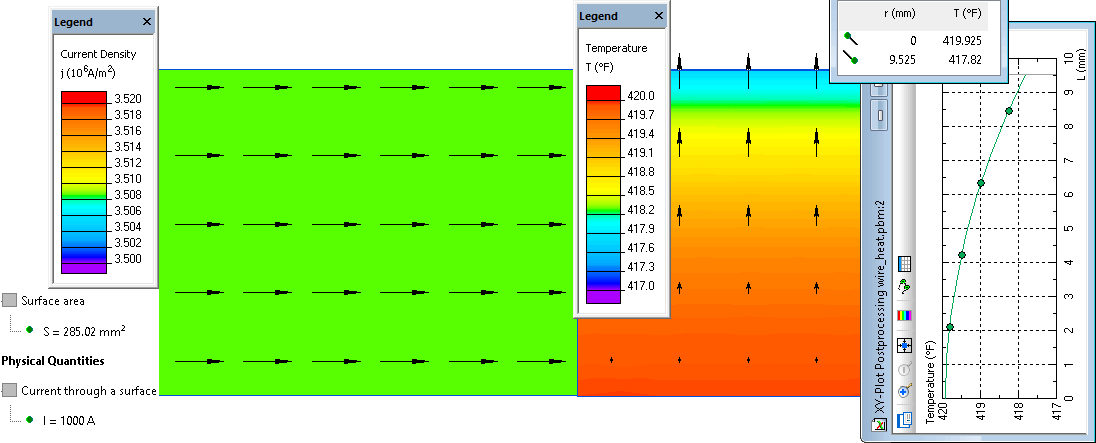QuickField

A new approach to field modelling

Main >> >> Temperature distribution in an electric wire

# Temperature distribution in an electric wire

QuickField simulation example

Calculate the temperature distribution in a long current carrying wire.

Problem Type
Axisymmetric multiphysics problem of DC Conduction coupled to Heat Transfer.

Geometry

Given
Wire diameter d = 0.75";
Resistance ρ = 9.35·10-8 Ohm/m;
Electric current I = 1000 A;
Thermal conductivity λ = 13 Btu·ft/(h·ft²·°F) = 22.5 W/(K·m);
Convection coefficient α = 5 Btu/(h·ft²°F) = 28.4 W/(K·m²);
Ambient temperature To = 70°F = 21.1°C.

Calculate the temperature distribution in the wire.

Solution
We arbitrary chose a 1-foot (12") piece of wire to be represented by the model.
Material electric conductivity is σ = 1/ρ = 1/9.35e-8 = 10.7·106 S·m.
For the conduction problem we specify normal current density at one end j = I /(πd²/4) [A/m²] and zero voltage at another end of the wire, and a zero current condition at its surface.
For heat transfer problem we specify zero thermal flux conditions at the sections of the wire and a convection boundary condition at its surface.

Results

 Reference* QuickField Center line temperature 419.9°F 419.9°F Surface temperature 417.9°F 417.8°F

Electric current density and temperature distribution in an electric wire:*Reference: W. Rohsenow and H. Y. Choi, "Heat, Mass, and Momentum Transfer", Prentice-Hall, N.J., 1963.